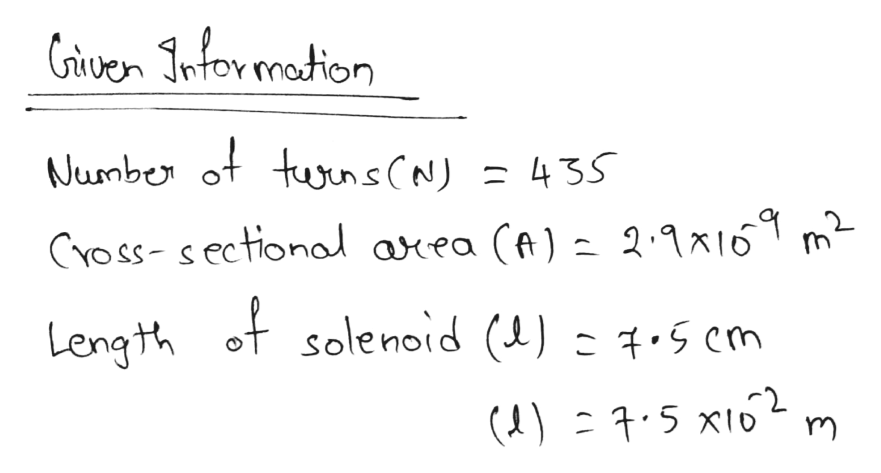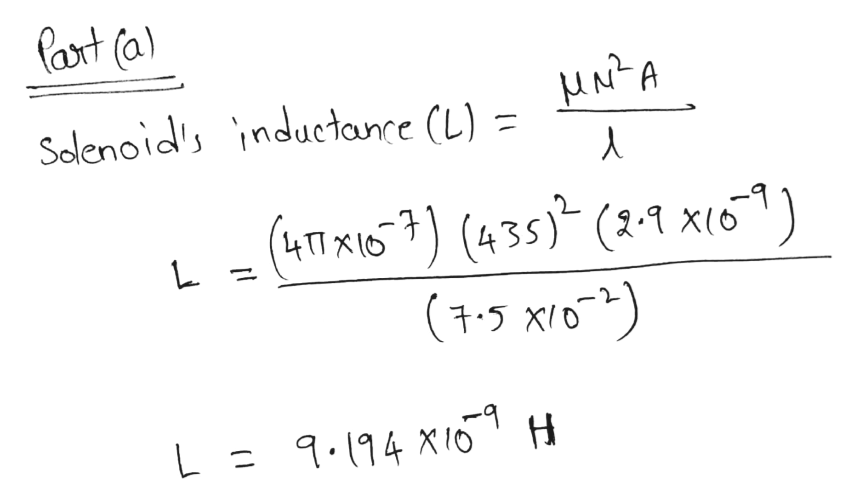# A solenoid with 435 turns has a length of 7.50 cm and a cross-sectional area of 2.90 ✕ 10−9 m2. Find the solenoid's inductance and the average emf around the solenoid if the current changes from +3.00 A to −3.00 A in 7.33 ✕ 10−3 s.  (a)the solenoid's inductance (in H) (b)the average emf around the solenoid (in V)

Question
9 views
A solenoid with 435 turns has a length of 7.50 cm and a cross-sectional area of 2.90 ✕ 10−9 m2. Find the solenoid's inductance and the average emf around the solenoid if the current changes from +3.00 A to −3.00 A in 7.33 ✕ 10−3 s.

(a)
the solenoid's inductance (in H)

(b)
the average emf around the solenoid (in V)
check_circle

Step 1help_outlineImage TranscriptioncloseCiven Intor motion Number of tuunsCN) = 435 CYOSS-sectional axea (A) 2.1x16 of solenoid (L) - 1.5 cm Length fullscreen
Step 2help_outlineImage TranscriptionclosePart (a) Solenoid's inductance (L) = (41 x16 (435) (2-1 x16"1) (+5 X0) 9.194 XIO fullscreen

### Want to see the full answer?

See Solution

#### Want to see this answer and more?

Solutions are written by subject experts who are available 24/7. Questions are typically answered within 1 hour.*

See Solution
*Response times may vary by subject and question.
Tagged in

### Electromagnetic Induction